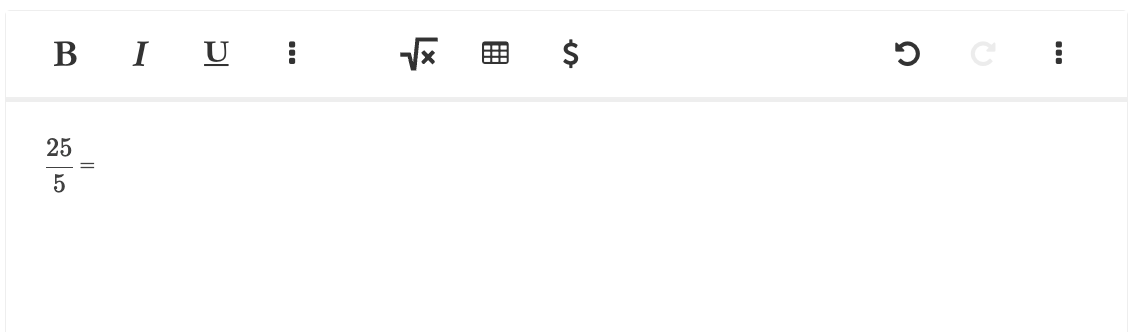### Write expressions/equation editor (math)

• Enables the student to construct equations using mathematical symbols in an equation editor.

The function is found in the writing area for the students.< Back to list of available tools/accommodations